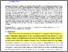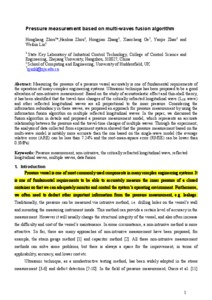Zhou, Hongliang, Zhou, Hanhua, Zhang, Hongjian, Ge, Xiaocheng, Zhao, Yanjie and Lin, Weibin (2017) Pressure measurement based on multi-waves fusion algorithm. IET Science, Measurement & Technology, 11 (3). pp. 354-362. ISSN 1751-8830
Abstract

Measuring the pressure of a pressure vessel accurately is one of fundamental requirements of the operation of many complex engineering systems. Ultrasonic technique has been proposed to be a good alteration of non-intrusive measurement. Based on the study of acoustoelastic effect and thin-shell theory, it has been identified that the travel-time changes of the critically refracted longitudinal wave (LCR wave) and other reflected longitudinal waves are all proportional to the inner pressure. Considering the information redundancy in these waves, we proposed an approach for pressure measurement by using the information fusion algorithm on multiple reflected longitudinal waves. In the paper, we discussed the fusion algorithm in details and proposed a pressure measurement model, which represents an accurate relationship between the pressure and the travel-time changes of multiple waves. Through the experiment, the analysis of data collected from experiment system showed that the pressure measurement based on the multi-wave model is notably more accurate than the one based on the single-wave model (the average relative error (ARE) can be less than 7.24% and the root-mean-square error (RMSE) can be lower than 0.3MPa).

Information
Library
DocumentsPreview
Pressure measurement based onmulti-waves fusion algorithm2016-10-22.pdf - Accepted Version